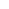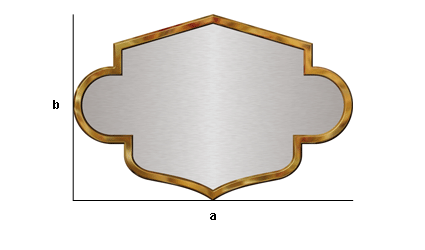Free Samples Distributors FAQ ScaleguidebookmarkHere you can calculate fast and simply the scaling factor of your graphic. Fill the original side length of your graphic into "Side A" and the new length into "scale to". The final value of the scaling factor is indicated immediately. After that you can calculate the height of your graphic. Fill the original height into "Side B" and the value of the height is indicated to you. Side A Scale to scaling factor - % Side B Scale to mm to inch Here you can convert some dimensions for example mm to inch. from to mm cm inch mm cm inch Calculator Here you can calculate rough values. Enter two existing values and the remaining values will be calculated. Input Result Side a Side b Diagonal Surface Extent